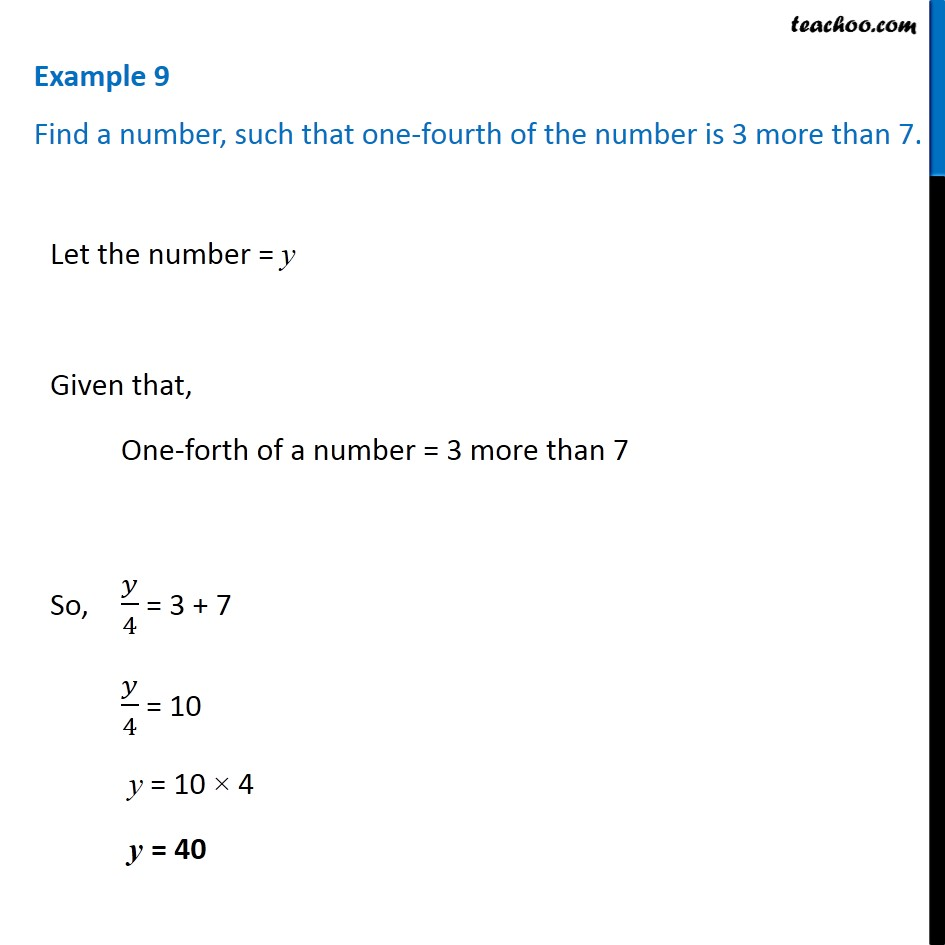Making equations from statements and solving

Chapter 4 Class 7 Simple Equations
Concept wiseIntroducing your new favourite teacher - Teachoo Black, at only ₹83 per month

### Transcript

Example 9 Find a number, such that one-fourth of the number is 3 more than 7. Let the number = y Given that, One-forth of a number = 3 more than 7 So, 𝑦/4 = 3 + 7 𝑦/4 = 10 y = 10 × 4 y = 40